Gaussian overlap model

The Gaussian overlap model was developed by Bruce J. Berne and Philip Pechukas  and is given by Eq. 3 in the aforementioned reference: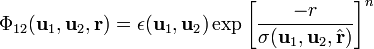$\Phi_{12}(\mathbf{u}_1,\mathbf{u}_2,\mathbf{r}) = \epsilon(\mathbf{u}_1,\mathbf{u}_2) \exp \left[ \frac{-r}{\sigma (\mathbf{u}_1,\mathbf{u}_2, \hat{\mathbf{r}}) } \right]^n$

where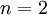$n=2$,$\Phi_{12}(r)$ is the intermolecular pair potential,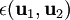$\epsilon(\mathbf{u}_1,\mathbf{u}_2)$ and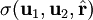$\sigma (\mathbf{u}_1,\mathbf{u}_2, \hat{\mathbf{r}})$ are angle dependent strength and range parameters, and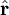$\hat{\mathbf{r}}$ is a unit vector. Not long after the introduction of the Gaussian overlap model Stillinger  proposed a stripped-down version of the model, known as the Gaussian core model. For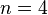$n=4$ a Soft cluster crystal phase has been observed. For Note that as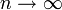$n \rightarrow \infty$ this potential becomes the penetrable sphere model.

Phase diagram

The phase diagram of the Gaussian-core model has been calculated by Prestipino et al. while the solid-liquid phase equilibria has been calculated by Mausbach et al  using the GWTS algorithm.

.